# The General Linear Model

I’m not a fan of how introductory statistics is taught, in decision-tree cookbook fashion where students have to memorize which analysis is most appropriate for which circumstance. I think a much better way to teach statistics (and a much better way to think of it) is to teach the general linear model (GLM). GLM doesn’t care whether there are one or two predictor variables, whether the variables are quantitative or qualitative, or the number of levels per variable. All it cares about is which variable is the outcome and which are the predictors. (And GLM can even handle multiple outcomes as well!)

Methodologists, as far as I know, have no “name” for an analysis with two categorical predictors and two quantitative predictors. Instead, we simply plug those variables in to the equation:

\(Y = A + B + X + Z\)

where A/B are categorical and Z/X are numeric. The computer (and the mathematics) don’t care what we call it and the computer doesn’t require a decision tree (other than one that specifies which predictors are numeric and which are categorical).

Shouldn’t plotting be like that as well? Shouldn’t we just have to tell a computer our outcome variable (i.e., what’s on the Y axis) and what predictor variables we have? Then shouldn’t the computer figure out for us how to plot it?

That’s the idea behind flexplot. Flexplot is a common language for plotting where the user simply specifies what the outcome is and what the predictors are. The computer then decides the optimal way of plotting the variables. However, the user does has flexibility to decide which variables are going to be on the X axis, which will be plotted as separate lines/symbols/colors, and which will be panelled.

In this article, I’m going to show you how to use flexplot to graph the most common sorts of analyses in psychology.

## Preliminaries

I’m just going to load a dataset that I’m going to use throughout this post. I made these data up a few years ago and they’re good for showing just about any sort of analysis you might be interested in. Essentially, it simulates data where participants were randomly assigned to different therapies for weight loss (behaviorist versus cognitive therapy). The dataset also contains other variables, such as motivation scores and income.

So with that, let’s load the fifer package, as well as the exercise datasets:

## Independent T-Test/ANOVA

In this situation, we have a grouping variable (e.g., treatment versus control, male versus female, low/medium/high medication) and we want to see how scores on the dependent variable vary as a function of group. To do so, we can use a “median dot plot” as I call them (or a mean dot plot, if you choose to report the mean instead of the median). The median is shown as a large red dot, along with interquartile ranges. I prefer nonparametric versions (i.e., medians/IQRs) rather than means/standard errors, just in case the data are not normally distributed. The “scores” for the rewards/no-rewards conditions have been “jittered,” which just means that noise has been added so they don’t overlap as much.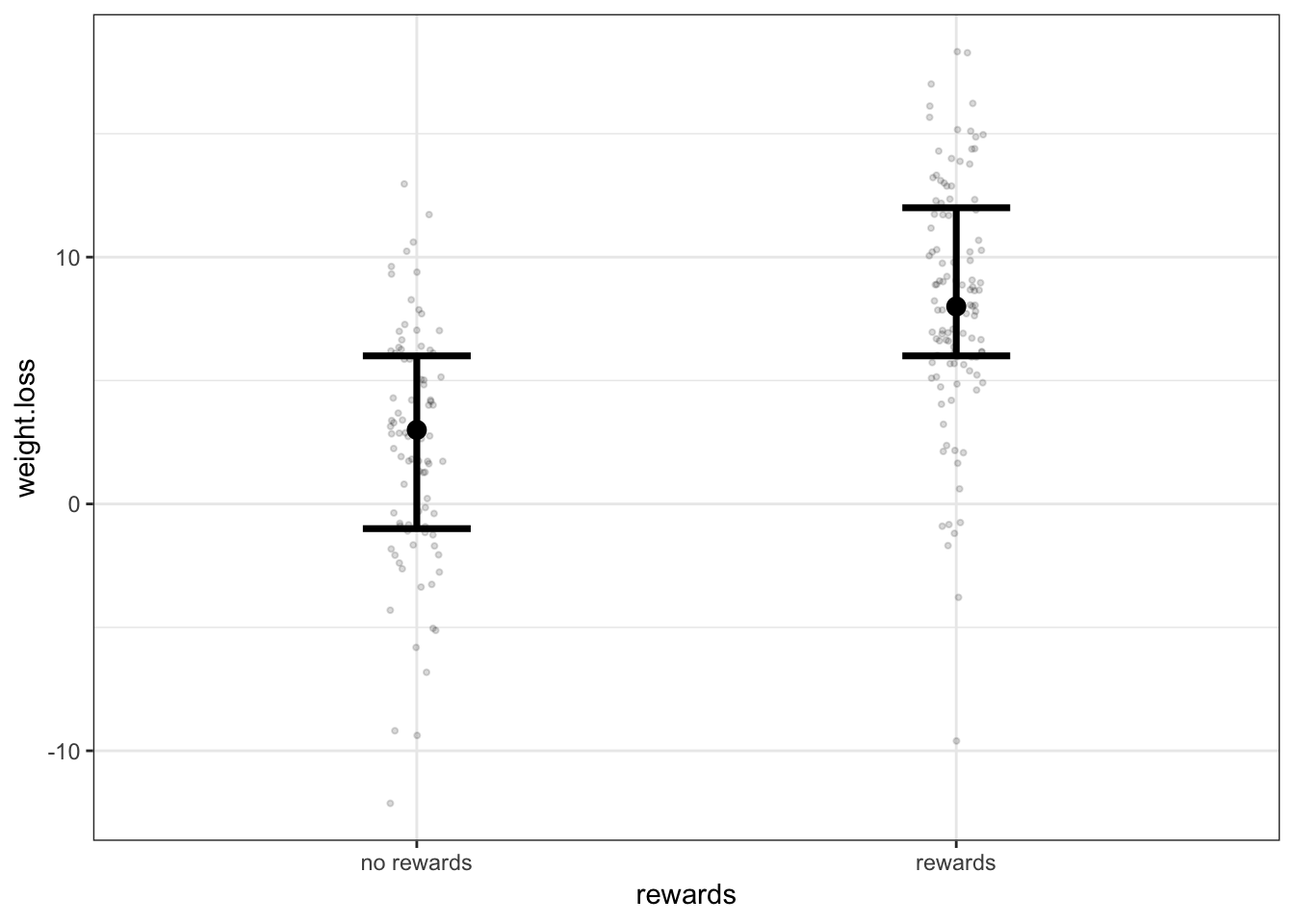## Simple Regression

The clear “winner” for graphing simple regression is the scatterplot. One nuance I like to add (at least in the early stages of investigation) is to overlay a lowess line, rather than a regression line. A lowess line will “bend” with the data if it feels the need to bend, which prevents my eye from overlooking evidence of nonlinearity.

To do so in flexplot, one simply types: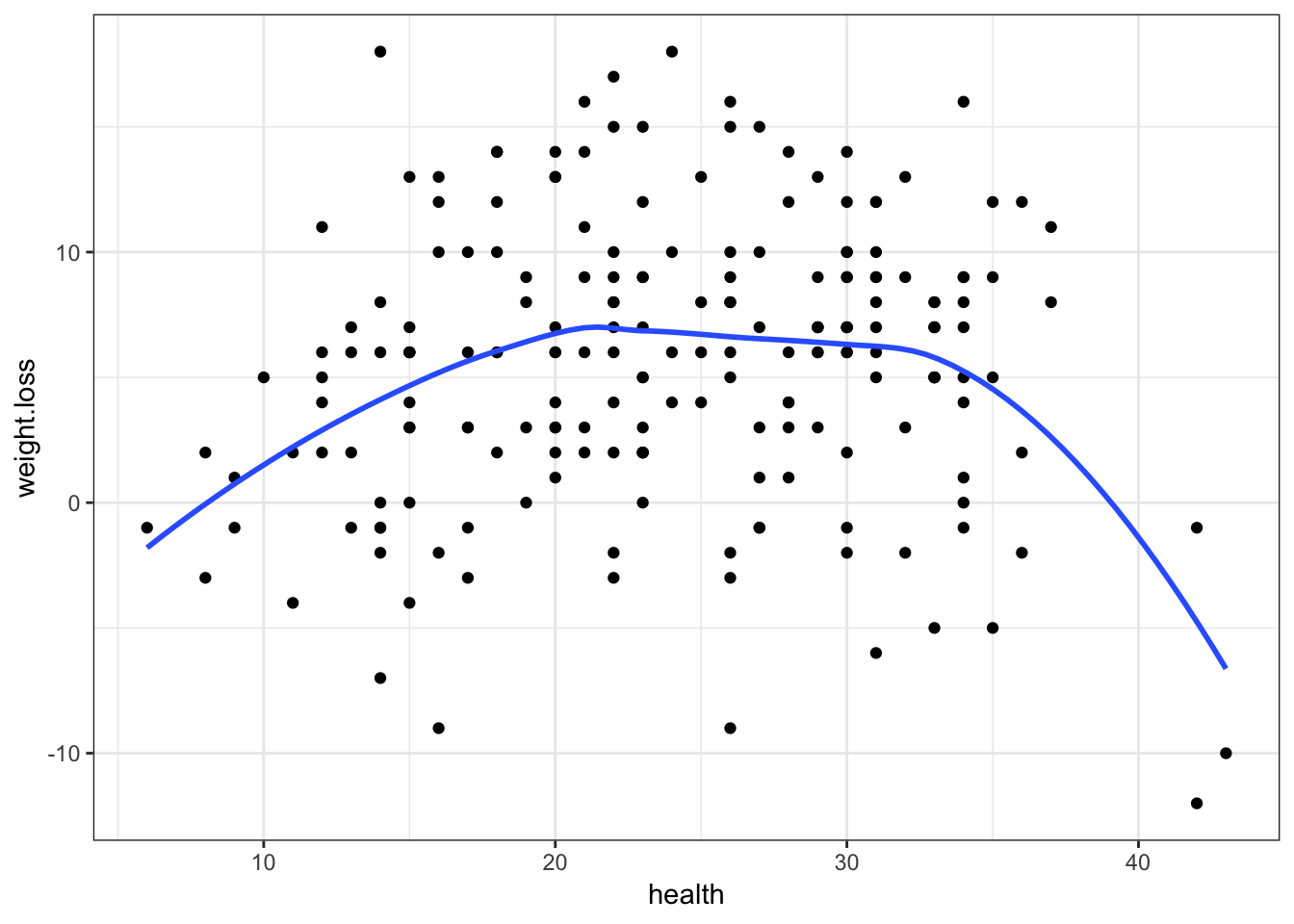Notice the above graphic does bend. Good thing we plotted the lowess line!

## ANCOVA

Before demonstrating how to plot an ANCOVA, it is necessary to begin with a little theoretical backgound. Recall that an ANCOVA attempts to determine whether groups (e.g., treatment versus control) differ on an outcome variable, after controlling for a continuous measure. This becomes a little tricky because we then have to represent graphically what it means to “control” for another variable. One way to do this is to do a simple regression between the outcome and the covariate, export the residuals, then plot the grouping variable on the X axis and the residuals on the Y axis. Unfortunately, this mucks up the scale of the Y axis (it will be centered at zero) and makes it harder to interpret. To fix that, I like to add the mean of the outcome back into the residuals These plots are called “Added Variable Plots” (though added variable plots don’t usually add the mean back in like I do).

But, if you’re doing this in R, you don’t have to worry about all that. Just use the added.plot function: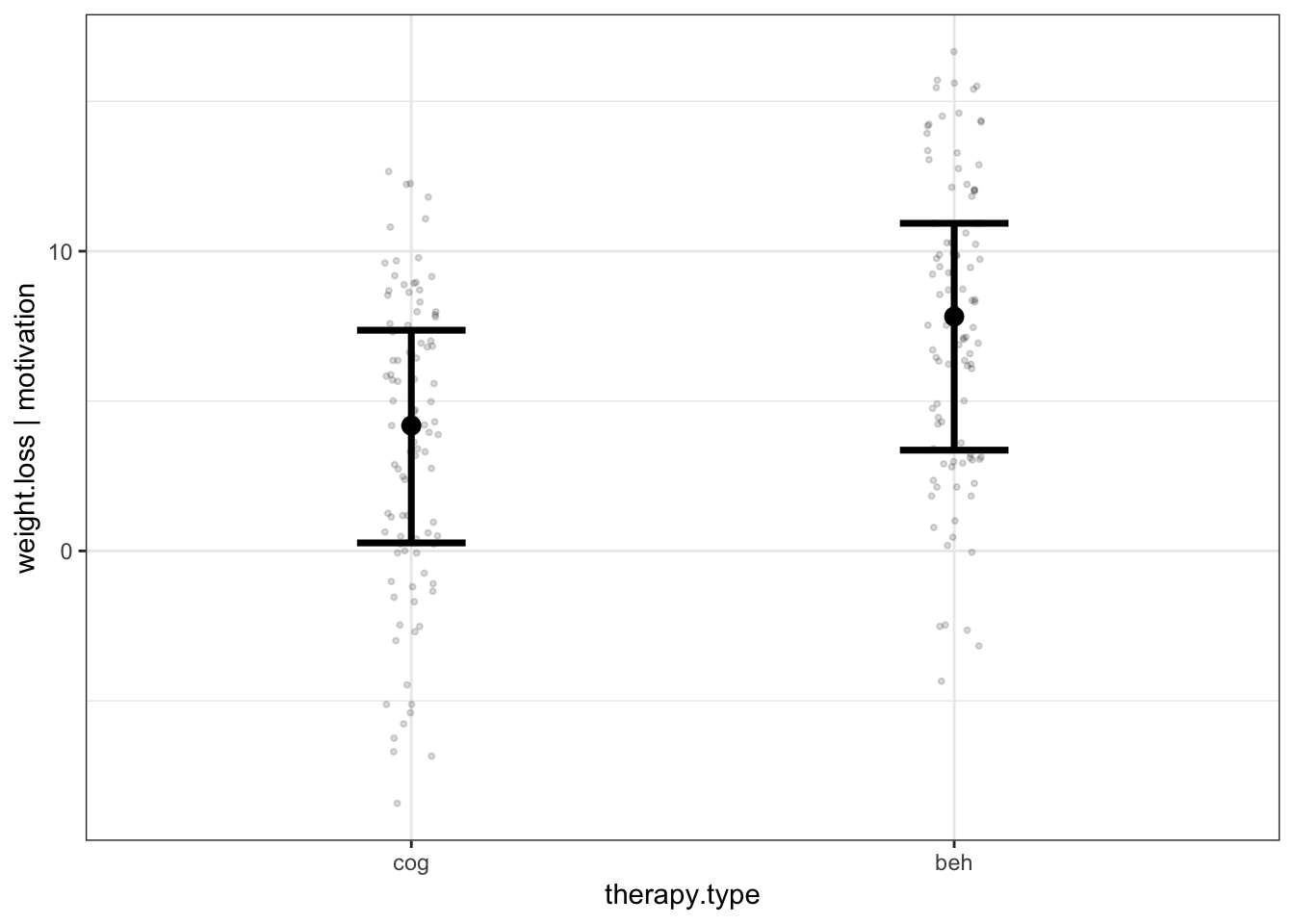Note that this function will control for the first listed variable (motivation in this case).

One limitation of added variable plots is they assume the covariate is linear and does not interact with the grouping variable. To check that this is the case, it’s best to plot the groups as separate lines and put the covariate on the X axis, as follows: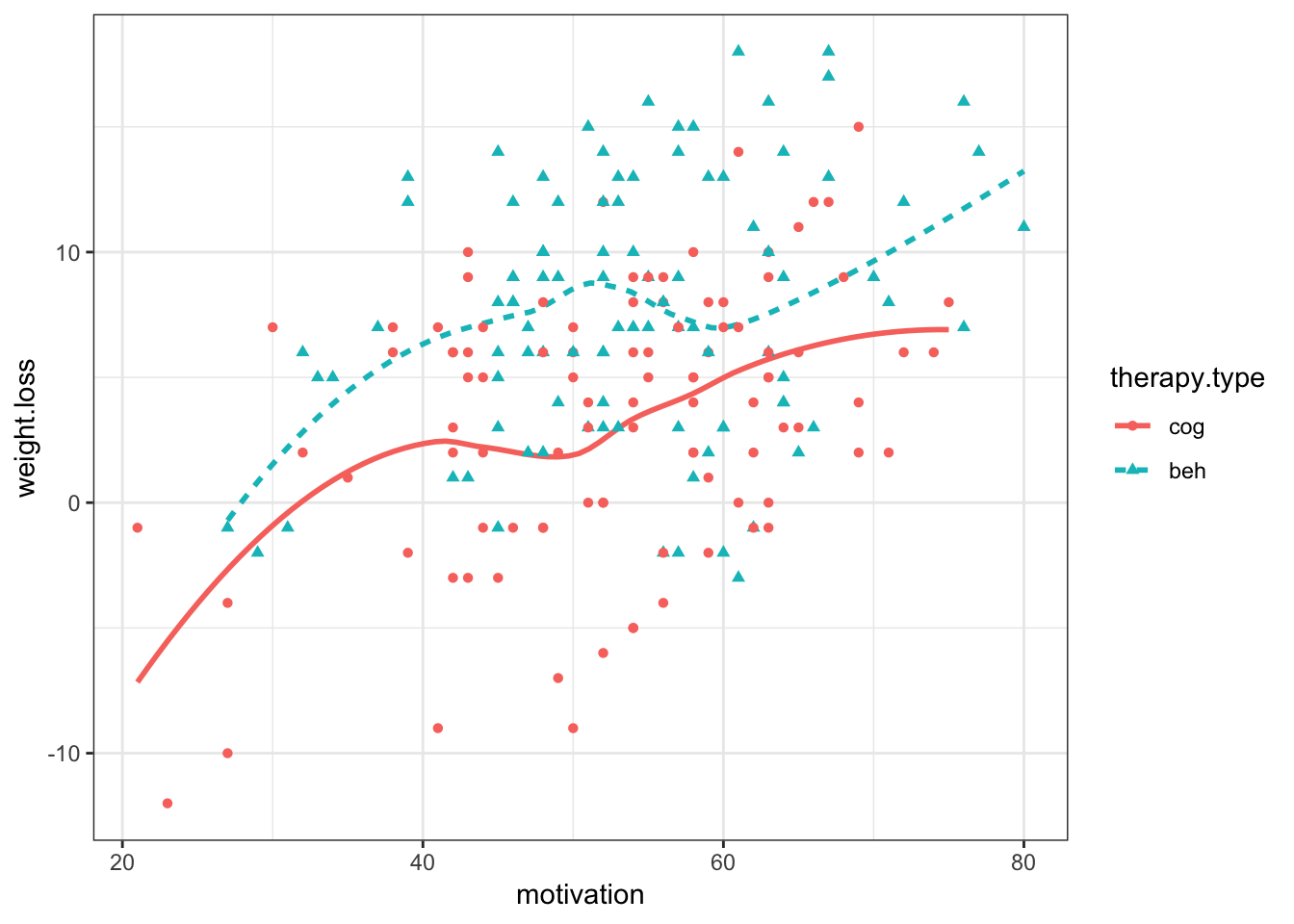Or put the lines in separate panels: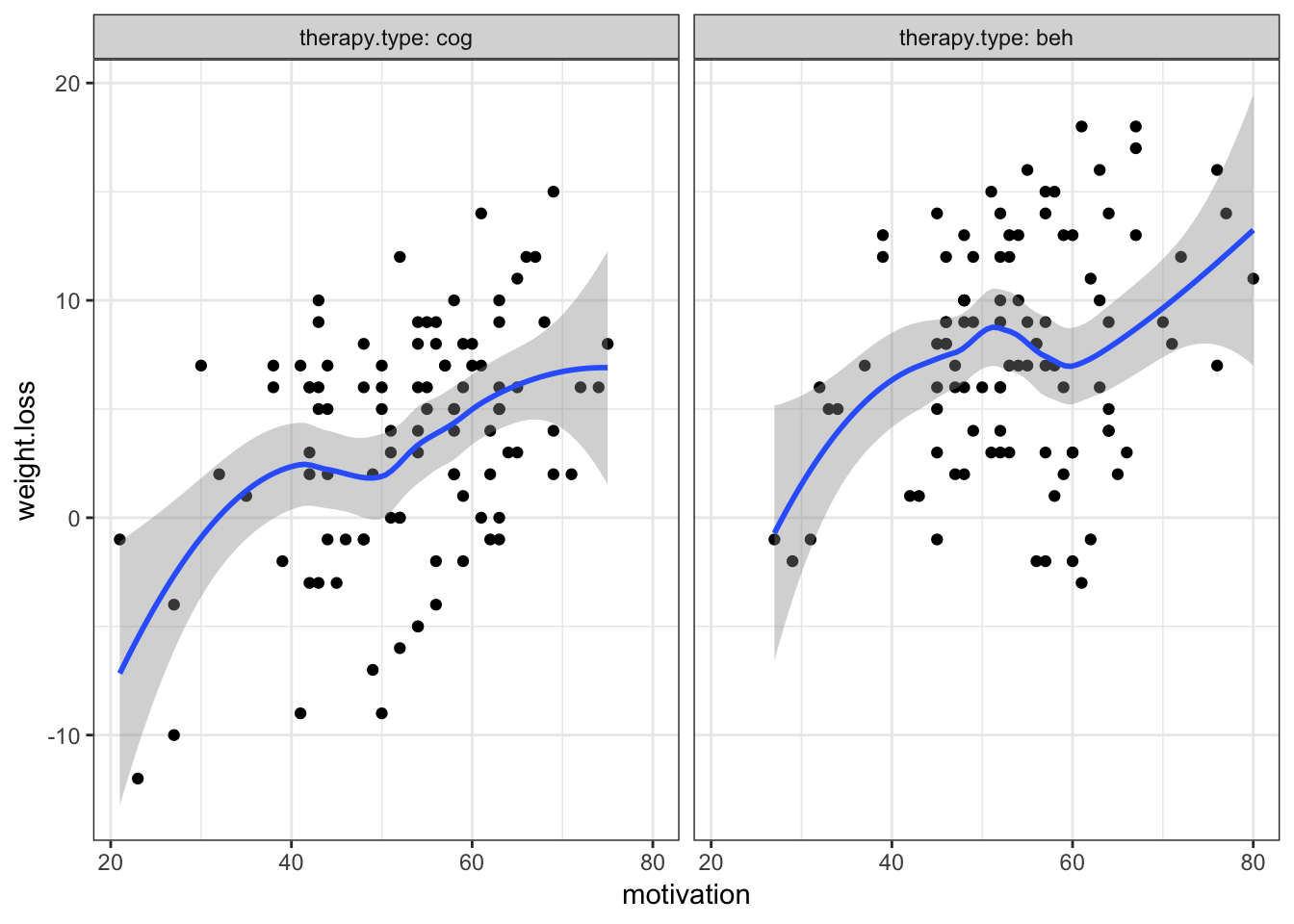Notice the notation: to panel a variable, put it to the right of a “|”. Otherwise, categorical variables show up as different lines.

In both cases, there’s a little “bendiness,” but not enough to worry about.

An important “take home” message from these three plots is this: if an analysis can be plotted multiple ways, plot it all ways. Each way provides a different “perspective” that may yield greater insight.

## Factorial ANOVA

Recall a Factorial ANOVA has two IVs rather than just one and it allows us to assess the strength of an interaction between two categorical variables. This is quite easy to do in flexplot: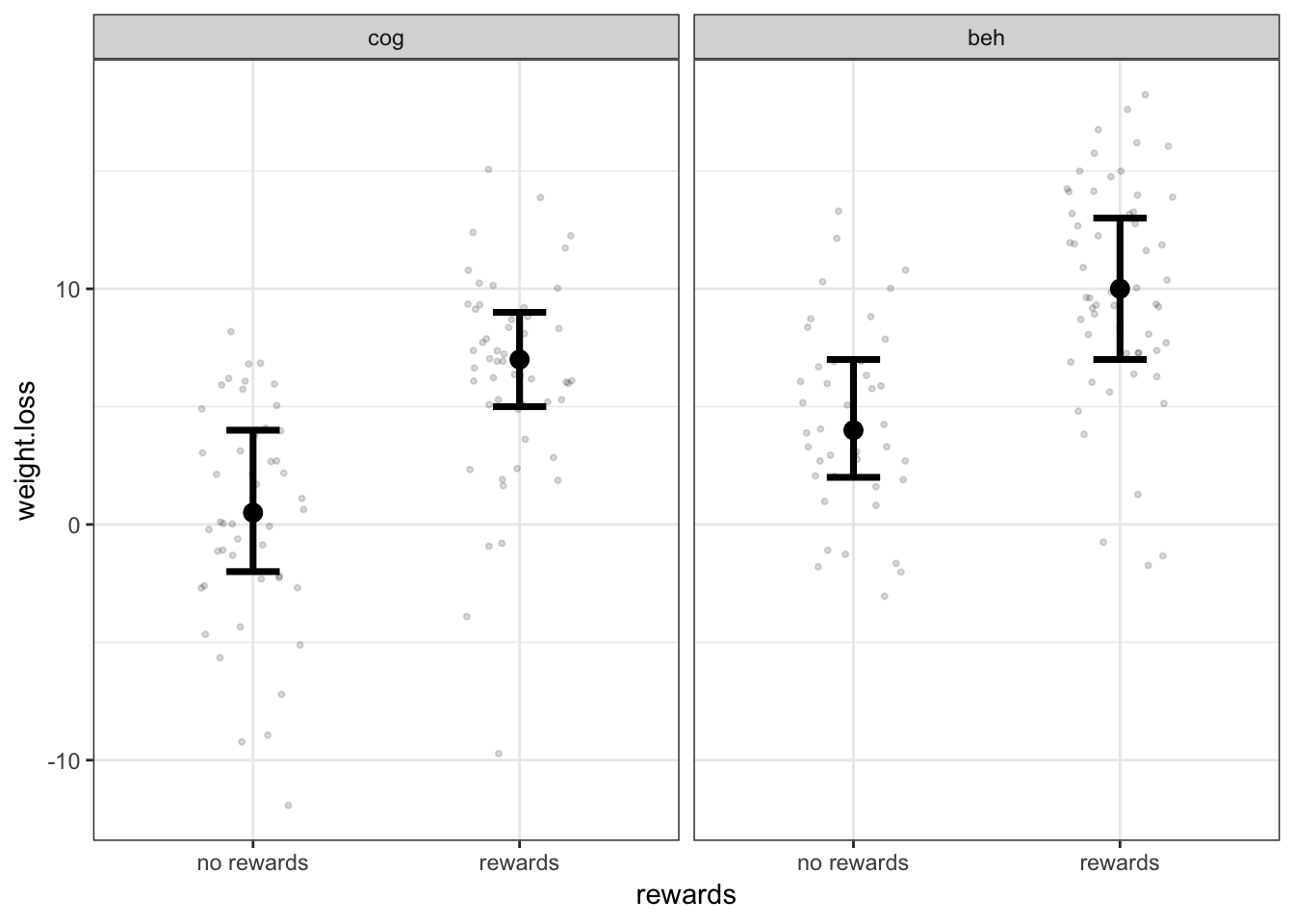It is common to plot “interaction plots” when we have two categorical predictors and attempt to visually inspect the degree to which the two lines deviate from parallel. I always favor plotting the raw data in addition to the numeric summaries (such as the mean/standard deviation). For this reason, I prefer to show the lines in two separate panels to minimize overlap.

You could also plot them in the same panel: